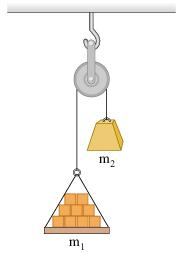# Problem: A load of bricks with mass = 15.0 kg hangs from one end of a rope that passes over a small, frictionless pulley. A counterweight of mass = 27.6 kg is suspended from the other end of the rope, as shown in the figure. The system is released from rest.1) What is the magnitude of the upward acceleration of the load of bricks?2) What is the tension in the rope while the load is moving?

###### FREE Expert Solution

1)

Newton's second law:

$\overline{){\mathbf{\Sigma }}{\mathbf{F}}{\mathbf{=}}{\mathbf{m}}{\mathbf{a}}}$

This problem involves a system of objects. When solving this type of problem, the tension in the rope is assumed to be uniform. The magnitude of the acceleration of the system is equal on both sides.

87% (238 ratings)###### Problem DetailsA load of bricks with mass = 15.0 kg hangs from one end of a rope that passes over a small, frictionless pulley. A counterweight of mass = 27.6 kg is suspended from the other end of the rope, as shown in the figure. The system is released from rest.

1) What is the magnitude of the upward acceleration of the load of bricks?

2) What is the tension in the rope while the load is moving?